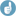## CI inclusion ≠ TOST [BE/BA News]

Hi ElMaestro,

» does anyone know what this sentence means:

Yes.» "The calculation of the 90% confidence interval (CI) of the mean test/comparator ratio for the primary PK parameters‚…

$$\small{H_0:\frac{\mu_\textrm{T}}{\mu_\textrm{R}}\notin \left [ \theta_1, \theta_2 \right]\:vs\:H_1:\theta_1<\frac{\mu_\textrm{T}}{\mu_\textrm{R}}<\theta_2}\tag{1}$$

» … should not be confused with the two one-sided t-tests employed to reject the null hypothesis of non-equivalence…

$$\small{H_\textrm{0L}:\frac{\mu_\textrm{T}}{\mu_\textrm{R}} \leq \theta_1\:vs\:H_\textrm{1L}:\frac{\mu_\textrm{T}}{\mu_\textrm{R}}>\theta_1}\tag{2a}$$ $$\small{H_\textrm{0U}:\frac{\mu_\textrm{T}}{\mu_\textrm{R}} \geq \theta_2\:vs\:H_\textrm{1U}:\frac{\mu_\textrm{T}}{\mu_\textrm{R}}<\theta_2}\tag{2b}$$
» … The end result is the same, but these are not the same calculations."Exactly!For decades global guidelines ask for for the confidence interval inclusion approach $$\small{(1)}$$. In Schuirmann’s famous TOST procedure $$\small{(2)}$$ one gets two p-values; one for $$\small{(2\textrm{a})}$$ and another one for $$\small{(2\textrm{b})}$$. Nothing else. BE is concluded if both Nulls are rejected.
I think that statisticians of the WHO are fed up reading in $$\frac{\mathfrak{protocols}}{\mathfrak{reports}}$$ …

Bioequivalence $$\frac{\textrm{will be}}{\textrm{has been}}$$ assessed by the Two-One-Sided Tests procedure (Schuirmann 1987).

… only to find the 90% CI in the report.
BTW, only once I have seen TOST performed (in 1991). Lead to a deficiency letter: “The applicant should provide the 90% CI.”

Chow and Liu1 erred when stating

The two one-sided t tests procedure is operationally equivalent* to the classic (shortest) confidence interval approach; that is, if the classic (1–α)×100% confidence interval for µTµR is within (θL, θU), then both H01 and H02 are also rejected at the α level by the two one-sided t tests procedure.

I would not go that far like Brown et al.2 stating that

This similarity [between level α TOSTs and a 1–2α CI] is somewhat of a fiction, based more on an algebraic coincidence rather than a statistical equivalence.
[my insert]

More details given by Berger and Hsu.3 Already in the abstract:

The misconception that size-α bioequivalence tests generally correspond to 100(1–2α)% confidence sets […] lead[s] to incorrect statistical practices, and should be abandoned.

When reviewing stuff I insist in deleting the – all too common – TOST-statement as well (i.e., claiming $$\small{(2)}$$ whilst performing $$\small{(1)}$$).

• I never understood what “operationally equivalent” means. Technically calculating 2 one sided t-tests is not the same as a two-sided 90% CI. Or do they mean hitting once the models are set up in the preferred software?
You can’t really say “similar” if it’s the same again you want.
“Similar” means something different.
Anthony Burgess (Enderby Outside, 1968)

1. Chow S-C, Liu J-p. Design and Analysis of Bioavailability and Bioequivalence Studies. Boca Raton: Chapman & Hall / CRC Press; 3rd ed. 2009. p. 98.
2. Brown LD., Casella G, Hwang JTG. Optimal Confidence Sets, Bioequivalence, and the Limaçon of Pascal. J Am Stat Assoc. 1995;90(431):880–9. doi:10.2307/2291322.Open access.
3. Berger RL, Hsu JC. Bioequivalence Trials, Intersection–Union Tests and Equivalence Confidence Sets. Stat Sci. 1996;11(4):283–319. doi:10.1214/ss/1032280304.Open access.

Dif-tor heh smusma 🖖Helmut SchützThe quality of responses received is directly proportional to the quality of the question asked. 🚮
Science QuotesIng. Helmut Schütz